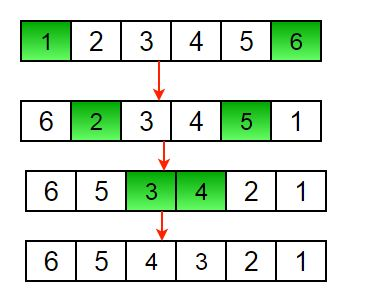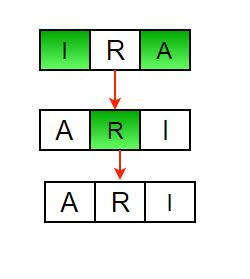GFG App
Open AppBrowser
Continue

# C Program to Reverse an Array or String

Given an array (or string), the task is to reverse the array/string.
Examples :

```Input  : arr[] = {1, 2, 3}
Output : arr[] = {3, 2, 1}

Input :  arr[] = {4, 5, 1, 2}
Output : arr[] = {2, 1, 5, 4}

```

Iterative way :

1) Initialize start and end indexes as start = 0, end = n-1
2) In a loop, swap arr[start] with arr[end] and change start and end as follows :
start = start +1, end = end – 1Another example to reverse a string:Below is the implementation of the above approach :

## C

 `// Iterative C program to reverse an array ` `#include ` ` `  `/* Function to reverse arr[] from start to end*/` `void` `rvereseArray(``int` `arr[], ``int` `start, ``int` `end) ` `{ ` `    ``int` `temp; ` `    ``while` `(start < end) ` `    ``{ ` `        ``temp = arr[start];    ` `        ``arr[start] = arr[end]; ` `        ``arr[end] = temp; ` `        ``start++; ` `        ``end--; ` `    ``}    ` `}      ` ` `  `/* Utility that prints out an array on a line */` `void` `printArray(``int` `arr[], ``int` `size) ` `{ ` `  ``int` `i; ` `  ``for` `(i=0; i < size; i++) ` `    ``printf``(``"%d "``, arr[i]); ` ` `  `  ``printf``(" ` `"); ` `}  ` ` `  `/* Driver function to test above functions */` `int` `main()  ` `{ ` `    ``int` `arr[] = {1, 2, 3, 4, 5, 6}; ` `    ``int` `n = ``sizeof``(arr) / ``sizeof``(arr);  ` `    ``printArray(arr, n); ` `    ``rvereseArray(arr, 0, n-1); ` `    ``printf``("Reversed array is  ` `"); ` `    ``printArray(arr, n);     ` `    ``return` `0; ` `} `

Output :

```1 2 3 4 5 6
Reversed array is
6 5 4 3 2 1

```

Time Complexity : O(n)
Recursive Way :

1) Initialize start and end indexes as start = 0, end = n-1
2) Swap arr[start] with arr[end]
3) Recursively call reverse for rest of the array.

Below is the implementation of the above approach :

## C

 `// Recursive C program to reverse an array ` `#include ` ` `  `/* Function to reverse arr[] from start to end*/` `void` `rvereseArray(``int` `arr[], ``int` `start, ``int` `end) ` `{ ` `   ``int` `temp; ` `   ``if` `(start >= end) ` `     ``return``; ` `   ``temp = arr[start];    ` `   ``arr[start] = arr[end]; ` `   ``arr[end] = temp; ` `   ``rvereseArray(arr, start+1, end-1);    ` `}      ` ` `  `/* Utility that prints out an array on a line */` `void` `printArray(``int` `arr[], ``int` `size) ` `{ ` `  ``int` `i; ` `  ``for` `(i=0; i < size; i++) ` `    ``printf``(``"%d "``, arr[i]); ` ` `  `  ``printf``(``"\n"``); ` `}  ` ` `  `/* Driver function to test above functions */` `int` `main()  ` `{ ` `    ``int` `arr[] = {1, 2, 3, 4, 5, 6}; ` `    ``printArray(arr, 6); ` `    ``rvereseArray(arr, 0, 5); ` `    ``printf``(``"Reversed array is \n"``); ` `    ``printArray(arr, 6);     ` `    ``return` `0; ` `} `

Output :

```1 2 3 4 5 6
Reversed array is
6 5 4 3 2 1

```

Time Complexity : O(n)

Please write comments if you find any bug in the above programs or other ways to solve the same problem.

My Personal Notes arrow_drop_up